×

Search anything:

# Syntax Directed Definitions

#### Compiler DesignSDDs specify values of attributes by associating the semantic rules of a programming language with the grammar productions. In this article, learn about SDDs, different types of attributes, and how SDDs are evaluated in a parse tree.

1. Introduction.
2. Inherited and synthesized attributes.
3. Evaluating SDDs at nodes of a parse tree.
4. Examples.
5. Summary.
6. References.

## Introduction.

A Syntax-directed definition is a context-free grammar with rules and attributes. Attributes are associated with the grammar's symbols. Rules are associated with the production of the grammar.

For example, given X is a symbol and a its attribute, we write, X.a. This denotes the value a at a node in the parse-tree with the label X.

When we implement nodes of a parse tree by records and objects, Xs attributes are implemented by data fields in the records representing its nodes.
Attributes can be numbers, types, strings, references, tables, etc.

## Inherited and synthesized attributes.

We have the following two attributes for non-terminals;

• Inherited attributes, A non-terminal B at node N in a parse tree is defined by a semantic rule that is associated with the production at the parent of node N.
This production must have B as its symbol in its body. An inherited attribute at a node N is defined in terms of attribute values at the parent of N, N, and the siblings of N.

• Synthesized attributes, A non-terminal A at node N in a parse tree is defined by a semantic rule that is associated with the production at N.
This production must have A as its head. A synthesized attribute is defined in terms of values at the children of N and N itself.

## Evaluating SDDs at nodes of a parse tree.

Even though the translator does not need to build a parse tree, we use a parse tree to visualize that specified translation by an SDD.

We apply the rules of a Syntax Directed Definition after constructing the tree and then using rules to evaluate attributes at each of the nodes in the tree.
An annotated parse tree is a tree that shows the values of its attributes.

We need to construct and evaluate the attributes of an annotated parse tree. Before we evaluate a node in the tree, we should first evaluate all attributes upon which its value depends.

Using synthesized attributes we can evaluate attributes in a bottom-up order just like a post ordering traversal of the tree.

Given an SDD with both types of attributes, inherited and synthesized, we have no guarantee that there will be an order in which we evaluate node attributes.
For example, we have nonterminals A and B with synthesized and inherited attributes A.s and B.i respectively. We also have the following production rules;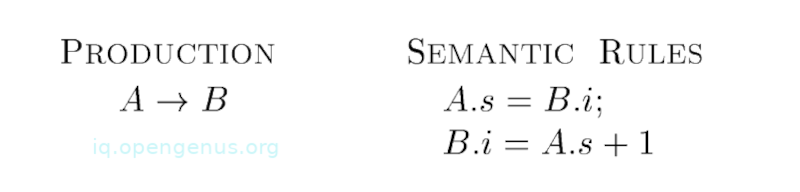These rules are circular and so it is impossible to evaluate either A.s at node N or B.i at the child of N without evaluating the other.
This circular dependency is shown below;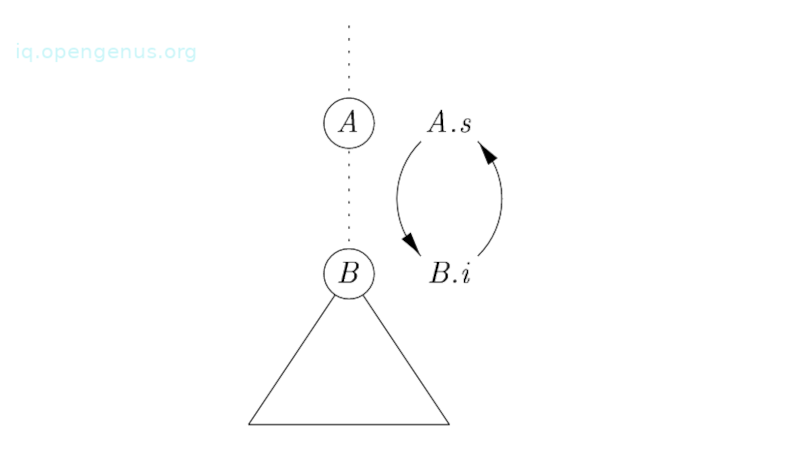It is computationally difficult to determine the existence of circularities in the parse tree that a given SDD could have to translate. However as we will learn here, there are useful subclasses of an SDD that is enough to guarantee that an order of evaluation exists.

## An example.

We have the following annotated parse tree for an input string 3 * 5 + 4n.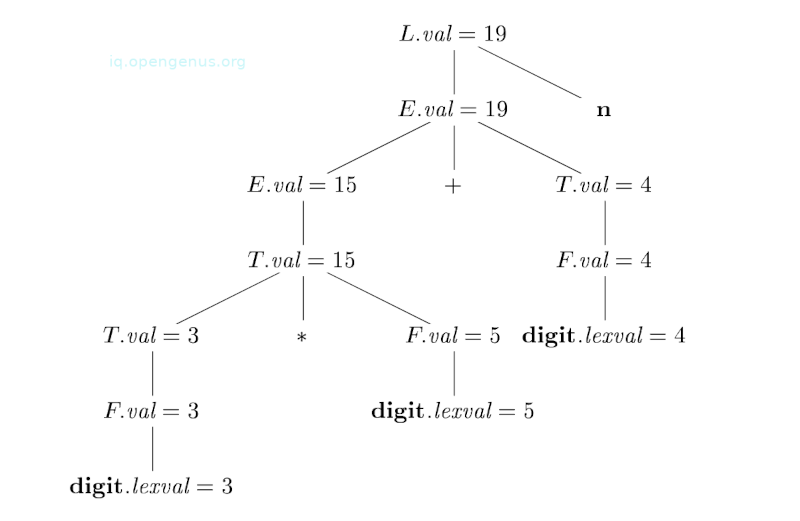It is constructed using the following grammar and rules.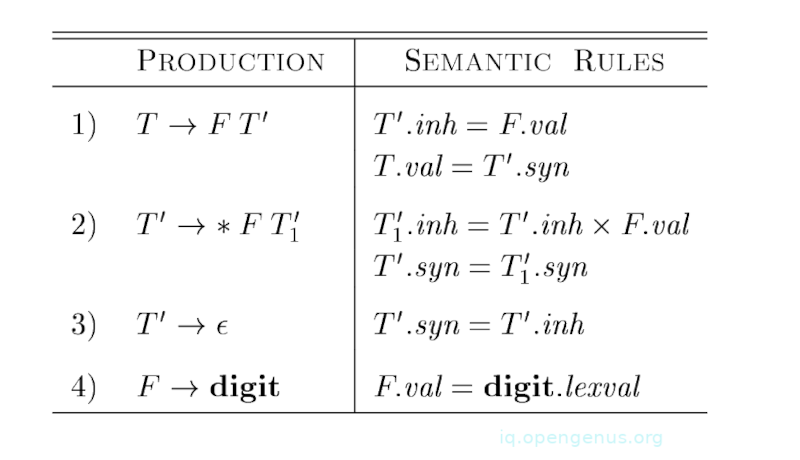We presume that lexval values are supplied by the lexical analyzer. Each node for the nonterminals has an attribute val that is computed in a bottom-up manner. The result is the association of values and nodes. For example, in the node with child *, after the computation between T.val = 3 and F.val = 5, we apply the rule stating that T.val is the product of these two values or 15.

We use inherited attributes when the structure of the parse tree does not match the abstract syntax of the source code.

Another example
We now see how inherited attributes can be used to overcome such mismatches due to a grammar designed for parsing rather than translation.
We have the following SDD the computes terms such as 3 * 5 and 3 * 5 * 7.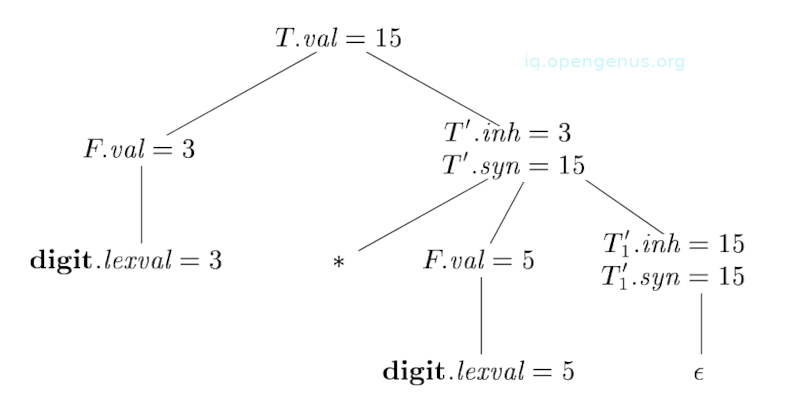The top-down parse of 3 * 5 starts with the production T -> FT'. In this case, F generates digit 3, and the operator * is generated by T'. Therefore, the left operand 3 appears in a difficult subtree of the parse tree from *.
An inherited attribute, therefore, is used to pass the operand to the operator.

In this example, the grammar is an excerpt from a non-left recursive version of the expression grammar.

Semantic rules are based on the idea that the left operand of the operator * is inherited. That is, the head T' of production T' -< F${\mathrm{T}}_{1}^{\text{'}}$ inherits the left operand of * in the production body.

Given x * y * z as the root of the subtree for * y * z inherits x. Then the root of the subtree for * z inherits the value of x * y and so forth.

Let's see how semantic rules are used by considering the following annotated parse tree for 3 * 5.

The left-most leaf in the tree labeled digit has attribute value lexval = 3 where 3 is supplied by the lexical analyzer.

Its parent is for the production 4, F -> digit.
The only semantic rule that is associated with this production defines F.val = digit.lexval that equals 3.

In the second child of the root, the inherited attribute T'.inh is defined by the semantic rule T'.inh = F.val that is associated with production1. Therefore the left operand 3 for * operator is passed from left to right across the children of the root.

The production at node T' is T' -> *F${\mathrm{T}}_{1}^{\text{'}}$. The inherited attribute ${\mathrm{T}}_{1}^{\text{'}}$.inh is defined by the rule ${\mathrm{T}}_{1}^{\text{'}}$.inh = 15. At the lower node for ${\mathrm{T}}_{1}^{\text{'}}$ the production ${\mathrm{T}}_{1}^{\text{'}}$ -> $ϵ$. The rule ${\mathrm{T}}_{1}^{\text{'}}$.syn = ${\mathrm{T}}_{1}^{\text{'}}$.inh defines ${\mathrm{T}}_{1}^{\text{'}}$.syn = 15. syn attribute pass the value 15 up the tree to node for T where T.val = 15.

## Summary.

A Syntax-directed definition is a context-free grammar with rules and attributes. Attributes are associated with the grammar's symbols. Rules are associated with the production of the grammar.

An annotated parse tree is a tree that shows the values of its attributes.
For non-terminals, we have two types of attributes, inherited and synthesized.

#### Erick Lumunge

Erick is a passionate programmer with a computer science background who loves to learn about and use code to impact lives positively.

Syntax Directed Definitions